# SBA Math - Grade 7: Circles Chapter Exam

Exam Instructions:

Choose your answers to the questions and click 'Next' to see the next set of questions. You can skip questions if you would like and come back to them later with the yellow "Go To First Skipped Question" button. When you have completed the practice exam, a green submit button will appear. Click it to see your results. Good luck!

### Page 1

#### Question 1 1. If angle AOB is 40 degrees, what is the measure of angle ACB?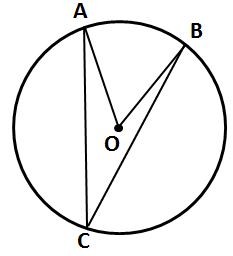### Page 2

#### Question 8 8. Mrs. Smith wants to build a semicircular garden, and she wants to surround it with a fence to keep out her dogs. She's measured half the garden's length at 6' long. How much fencing material will she need to buy? (Approximate pi to 3.14)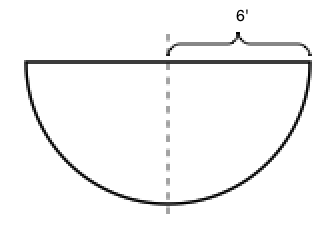#### Question 9 9. If angle OAB is 45 degrees, what is the measure of angle AOB?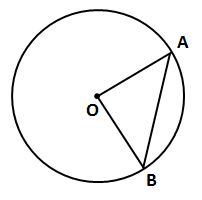### Page 3

#### Question 11 11. If angle ACB is 34 degrees, what is the measure of angle ADB?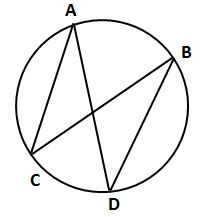#### Question 12 12. Which segment is a diameter of the circle?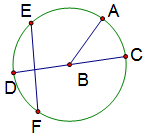#### Question 15 15. If angle ACB is 47 degrees, what is the measure of angle AOB?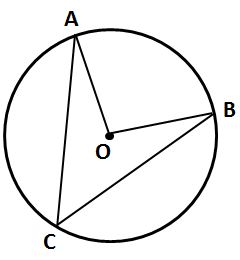### Page 4

#### Question 16 16. Although area and perimeter are measured in different units, what's the numerical difference between the area and perimeter of this semicircle? (Approximate pi to 3.14)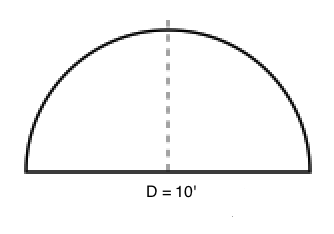### Page 5

#### Question 23 23. In the pictured circle, the measure of angle ACB is 22 degrees and the measure of angle BXD is 120 degrees. What is the measure of angle CAD?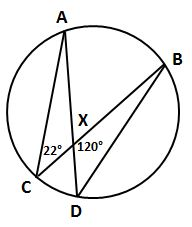### Page 6

#### SBA Math - Grade 7: Circles Chapter Exam Instructions

Choose your answers to the questions and click 'Next' to see the next set of questions. You can skip questions if you would like and come back to them later with the yellow "Go To First Skipped Question" button. When you have completed the practice exam, a green submit button will appear. Click it to see your results. Good luck!

Support